Next: Muon transfer Up: Muonic atom collisions Previous: Muonic atom collisions

### Elastic scattering

Elastic scattering processes dictate the deceleration of muonic atoms in the target, hence they determine the energy distribution at the time of the subsequent reaction (such as muon transfer or molecular formation) in the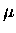CF cycle.

There is a relatively limited amount of experimental information on muonic atom scattering cross sections . In particular, there is hardly any data on energy dependent cross sections.

The elastic scattering cross section can be written in terms of the sum of the contributions of the partial waves: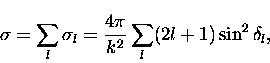(1)

where the physics is contained in the phase shift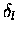. As one can see from Eq. 1.1, if for a given partial wave l,is approximately equal to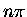(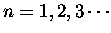), then the partial cross section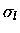goes to zero. For low energy collisions, where s wave (l=0) usually dominates, the total cross section can become very small, a process known as the Ramsauer-Townsend effect. If, on the other hand,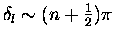, then the cross section becomes large and reaches a maximum value (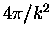) called the unitarity limit. It is interesting to note that all the phase shifts at the zero energy limit are fixed at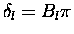by Levinson's theorem [25,26], where Bl is the number of three-body bound states of given l, illustrating the intricate connection between bound states and scattering properties. As we shall see it is the Ramsauser-Townsend effect that makes it possible to produce a muonic atom beam for our experiments.Next: Muon transfer Up: Muonic atom collisions Previous: Muonic atom collisions